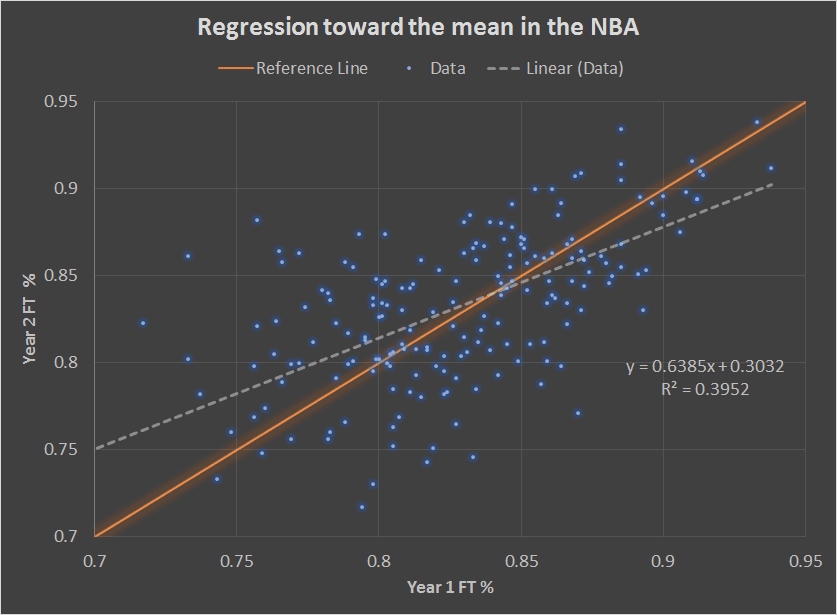## Friday, March 9, 2018

Published 10:10 PM by with 1 comment

# Regression Toward The Mean In The NBA

Regression toward the mean is a fascinating concept, and I went through some NBA data to test it...Free throw % from one year to the next

Regression toward the mean can take a long time to explain in full detail, so I'll jump straight to the example here. The plot at the top has free throw percentage for players from one year on the x-axis and free throw percentage from the same players from the next year on the y-axis. Each x, y point is the same player. The reference line will be explained below, and the equation is the linear fit to the data. The basic idea here is that part of the reason the best player in a given year is the best player is that he got lucky, so you'd expect him to be more average in the next year. Similarly, the worst player in a given year got unlucky so you'd expect him to be more average in the next year. This tendency to get more average when part of the result is due to chance is called regression toward the mean. You might have heard of this as the 'Madden curse' or the 'Sports Illustrated curse'.

A simple way to intuit this is to think about it in extremes. If the results are nothing but luck, there should be no pattern from year to year right? If it's a complete tossup, you would expect no relationship between year 1 and year 2. That would give you a slope of zero. For an example of this, imagine that instead of shooting free throws for the points, you flipped a coin. Similarly, if the results are nothing but skill, skill doesn't change that dramatically from year to year on average, so the slope should be very close to 1. If you assume that it's a combination of luck and skill, then the slope should be between 0 and 1. I added a reference line to the plot that has a slope of 1 and an intercept of zero that is the '100% skill with no change YOY' assumption.

Extending that logic then, you should expect a few things if you dig into the graph:
• if you go to the right of the average (~83% on the x-axis), you should see more points below the reference line than above it
• if you go to the left of the average, you should see more points above the reference line than below it
• if every player got better year to year on average (say they change the rules to make the shot easier), you should see all points biased above the reference line; if every player got worse, they should be biased below
• if skill is a significant component, you should generally see the highest y values to the right of the average and the lowest y values to the left
Another way of plotting this that is much more condensed but maybe easier to grasp is this:

All I've done there is group the data into three bins: <81% (worse than average), 81-85% (about average), and >85% (better than average). The y-axis is how much better the players in the bin performed in the next year.

1.